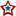#The Flipping Point Calculation

On the electoral table (as well as on the state graphs) I've added a flip percent column that lists the probability of a given state anointing the minority party of 2004 the 2008 victor. For example Bush won Iowa in 2004, but now the algorithm says there is a 99.75 percent chance of Iowa flipping into the Obama column for the 2008 Election. The probability is calculated using a Cumulative Distribution Function (CDF). The CDF computation begins with the most recent Local Regression result for the minority party of 2004 and the variance of that result; the algorithm then outputs the percent chance of the minority party of 2004 receiving the majority vote in 2008. The majority vote is calculated by adding the most recent Local Regression values for McCain and Obama and dividing by two.

In some cases where a state has just a single valid poll, the result incurs a zero divided by zero error; when this happens the output is normalized to be 0. Washington DC has no polls, thus making calculations difficult; to account for this I assume that DC will be a very large victory for the Democrats (as it has historically) and in turn a zero percent chance of flipping.

## 0 Response(s) to The Flipping Point Calculation(Word Verification)